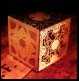View Single Post20-11-2009, 02:55 AM   #418
Senior Member

Join Date: Jan 2009
Location: ERY(UK)
Posts: 137
Likes: 1 (1 Post)Rodinometry and Gematria

Quote:
 Originally Posted by plainsightAnd here's something "new" to crack: The "magic square of the sun".http://www.redicecreations.com/speci...rowley666.html To understand why 666 is a magick number of the Sun, we must turn to the sacred teachings of the Hebrew Kabbalah where it is taught that the sphere of the Sun is the sixth emanation from the pure essence of God. To express this concept mathematically (something Kabbalists love to do) a square is composed of 36 squares (6 x 6). The numbers 1 to 36 are then arranged in a balanced way so that every row and every column add to the same number. That number is 111, and the sum of all the squares is 666." 666=9, 6*6=9
Here's something I made a couple of years ago which seems highly relevant to this example:There are similar dynamics here with 666 as total and 111 as axial lines through zero, as well as any pair of arbitrary rotationally symmetric triples also at 111.
Try adding a radially symmetric pair of the small numbers in the white triangles together and you get 111 every time, though the triples don't have to be adjacent for this to work - just radially/rotationally symmetric.
So it seems that any symmetric array of 6 tiles will sum to 111.

There are also arithmetic parallels each side of a vertical mirror axis, such that:

eg,

(4)+=(10)
(27)+=(33)

or,

(16)+=(35)
(2)+=(21)

How I arrived at this was actually indirectly from a coding of the Flower of Life based on the numbers 1-37 rather than 0-36.

The original website was a Geocities profile and I can't seem to find another example of that particular coding anywhere. It had an interesting property in that equilateral groups of 6 tiles summed to 114.

114 is the number of suras (chapters) in the Koran.
114 reduces to 6.
114=19x6.

I recall that "19" was the central number of that 1-37 coding pattern.
In the Koran it specifically states that: "Over it, is 19" [74:30].

The number "19" is also very important to the Bahai Faith - to which Rodin himself has an affiliation.

We are beginning to uncover the "gematria" basis (or perhaps extrapolation) of this stuff.
Rodin mentioned this in his video presentations, though I have not seen this demonstrated in detail anywhere before.

Another notable feature is the number 37.
In the above tiling, any tile and its associated opposing tile sum to 37. This seems to suggest that the central zero is also equal to 37, or at least its "other half" - intreging. If we take the zero to represent all of the grid, we'd have 37+666=703/37=19=>10=>1.

Here is a simple binary example of this "37" aspect:

000
001
010
011
100
101
110
111+
-----
= 444 => 444/ = 12

The result will always be a multiple of 37 for any 3-digit number.
(sum all permutations of a given 3-digit number)

eg, "147":

147
174
417
471
714
741+
-----
= 2664 => 2664/ = 72

The "Oracles of Zoroaster" may also be involved, like in this post I found on another forum.
This also ties in to the Solfeggio stuff too.

Remember that the Vector Equilibrium has 36 vectors - 24 edge vectors and 12 radial vectors.

With regard to relevance towards "Rodinometry", I would caution getting too bogged down into the various "gematria calculators" that can be found all over the web. My main focus with gematria is not necessarily the number values as such, but the underlying geometry of the metaconstructs involved. Once the correct constructural relationships are in place, then the numbers will take care of themselves.

So far with my article I am looking at combining Hebrew, Greek, Arabic, Tibetan and Sanskrit into a coherent system with Dogon Metaphysics as the underlying basis.